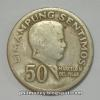#### You may also like### Thirty Nine, Seventy Five

We have exactly 100 coins. There are five different values of coins. We have decided to buy a piece of computer software for 39.75. We have the correct money, not a penny more, not a penny less! Can you discover what the five different types of coins are worth and how many of each we have saved?### History of Money

If you would like a new CD you would probably go into a shop and buy one using coins or notes. (You might need to do a bit of saving first!) However, this way of paying for the things you want did not always exist. Find out more ...### Debt Recovery

Tony and Tina can't work out which of them owes what to the other. Can you?

# Oh for the Mathematics of Yesteryear

##### Age 11 to 14 Challenge Level:

Correct solutions were received from Mary (Birchwood Community High School) and Chor Kiang. Well done.

### Part 1

First, find the total ounces of bread.
$600 \times35 \times24$ ounces $= 504000$ ounces

Now, find the new number of men-shares needed.
$4800 \times45 = 216000$

Divide $504000$ ounces by $216000$ to get $2\frac{1}{3}$ ounces a day.

Hence, each man must only eat $2\frac{1}{3}$ ounces of bread a day.

### Part 2

$£14 10\text{s} = 290\text{s}$

$£5 \; 8\text{s } 9 \text{d} = 108.75$ shillings

Weight (cwt)   Distance (miles) Amount (shillings)
$60$ can be transported for $20$ for $290$
$1$ can be transported for $20$ for $\frac{290}{60} = \frac{29}{6}$
$1$ can be transported for $30$ $\frac{29}{6}\times\frac{30}{20}= 7.25$
$\frac{1}{7.25}$ can be transported for $30$ $1$
$\frac{1}{7.25}\times 108.75$ can be transported for $30$ $108.75$

Since $\frac{108.75}{7.25} = 15$

$15$ cwt can be carried for $30$ miles at a cost of $£5 \; 8\text{s } 9 \text{d}$